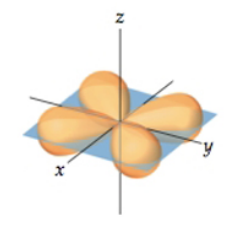# Problem: Part A. What is the angular-momentum quantum number (also called the azimuthal quantum number), ℓ, for the orbital shown here?Assign quantum numbers and predict characteristics of orbitals based on the contour representation.Wave functions provide information about an electron's probable location in space. This can be represented by an electron-density distribution diagram, called an orbital. An orbital is characterized by a size, shape, and orientation in space.

###### FREE Expert Solution
85% (424 ratings)View Complete Written Solution
###### Problem Details

Part A. What is the angular-momentum quantum number (also called the azimuthal quantum number), ℓ, for the orbital shown here?Assign quantum numbers and predict characteristics of orbitals based on the contour representation.

Wave functions provide information about an electron's probable location in space. This can be represented by an electron-density distribution diagram, called an orbital. An orbital is characterized by a size, shape, and orientation in space.